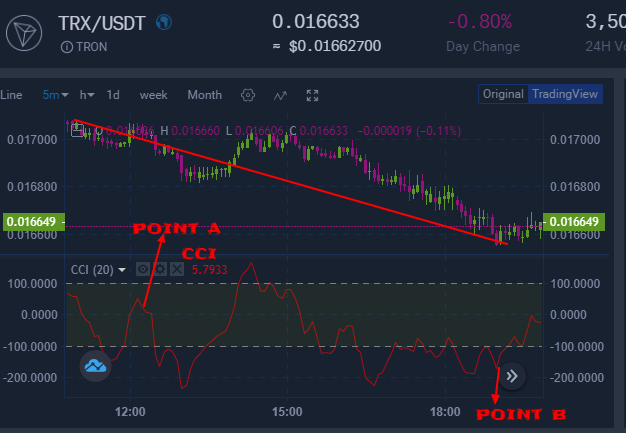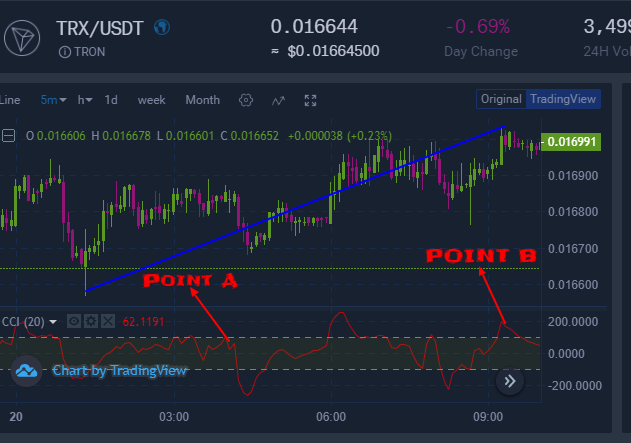Commodity channel index is an oscillator indicator.

As an oscillator indicator,Commodity channel index was developed by Donald Lambert to help traders measures the deviation of a commodity price from its average statistical price thus enabling them to know whether the market is in an overbought or oversold market condition.When the value of the commodity channel index is high,that is an indication  that the price is also high while when the value of the commodity channel index is low that is an indication that the price is also low.

These values are gotten by following the procedures as follows;

-Finding the typical price by adding the high,the low and the close price of each bar and then divide the whole result by 3.This is as follows;

TP=(HIGH+LOW+CLOSE)/3

-calculating the n-period SIMPLE MOVING AVERAGE of typical price.This is as follows;

SMA(TP,N)=SUM(TP,N)/N

-Subtraction SMA(TP,N) from typical prices of each of preceding n-periods.This is as follows;

D= TP-SMA(TP,N)

-Calculating the n-period SIMPLE MOVING AVERAGE of absolute D values.This is as follows;

SMA(D,N)=SUM(D,N)/N

-Multiplying the received SMA(D,N) by 0.015.This is as follows;

M=SMA(D,N)*0.015

-commodity channel index(CCI) is then gotten by dividing M by D.This is as follows;

CCI= M/D

Where as;

HIGH is maximum bar price

LOW is minimum bar price

CLOSE is close price

SMA is Simple Moving Average

N is number of periods used for calculation while;

SUM is the sum.

Commodity channel index oversold market

Since commodity channel index has an oscillation at point 0 where the CCI oscillates above or below, it therefore follows that When the CCI curve is below  0,that will be an indication that the cryptocurrency market is trending downwards thus the cryptocurrency trader should be trading in a downward market direction. On the other hand,when the CCI rises falls below -100(negative 100 ) that is an indication that there is an oversold  in the market thus the cryptocurrency trader should place a buy order of a given  amount of cryptocurrency  that he wants to start holding so that when the price of that bought cryptocurrency starts rising,his account will also start increasing in value. This is indicated as from the candlestick chart below;From the candlesticks chart above,there are two points,point A and point B. Point A represents the commodity channel index while point B represents the oversold point position.At point B,the commodity channel index has fallen below -100 thus an indication of an oversold condition at that point.This will signal the cryptocurrency trader to place a buy order of a given amount of TRX coin and start holding it. The cryptocurrency trader can either place a market buy order which will be executed instantly or can decide to place a limit order which will be executed when the market reaches that price.As soon as the price starts moving upwards,the account of cryptocurrency trader will start to increase in value in terms of USDT.

commodity channel index overbought market

Since commodity channel index has an oscillation at point 0 where the CCI oscillates above or below, it therefore follows that When the CCI curve is above  0,that will be an indication that the cryptocurrency market is trending upwards that he should be trading in an upward market direction. On the other hand,when the CCI rises above +100(positive 100 ) that is an indication that there is an overbought  in the market thus the cryptocurrency trader should place a sell order of the amount of cryptocurrency which he had previously purchased and was holding so that they can avoid making the account to decline in value. This is indicated as from the candlestick chart below;From the candlesticks chart above,there are two points,point A and point B. Point A represents the commodity channel index while point B represents the overbought point position.At point B,the commodity channel index has risen above +100 thus an indication of an overbought at that point.This will signal the cryptocurrency trader to place a sell order of the amount of TRX coin which he had previously bought and was holding by that time to avoid his account to start declining in value in terms of USDT when the price of TRX will start declining.

Recommendation: Cryptocurrency market is highly volatile. When you place a buy order, make sure to apply risk management to be safer incase your buy order goes against you.

You are always free to support our work from below;

skrill donation:[email protected]

Neteller donation:[email protected]

Ethereum; 0x346570c491b76c7cd51699bdd272762111b743a9

Litecoin: LeEH7B82ccXJ6QoibCryGJNpDGNx2x77fL

Dogecoin: DLShs2vqhMc1ggqo8MPPMBTcPAZdqCZoWd

lightning network:tipping.me:https://tippin.me/@quintomudigo